# Title 40

## SECTION 53.43

### 53.43 Test procedures.

§ 53.43 Test procedures.

(a) Sampling effectiveness - (1) Technical definition. The ratio (expressed as a percentage) of the mass concentration of particles of a given size reaching the sampler filter or filters to the mass concentration of particles of the same size approaching the sampler.

(2) Test procedure. (i) Establish a wind speed specified in table D-2 and measure the wind speed and turbulence intensity (longitudinal component and macroscale) at a minimum of 12 test points in a cross-sectional area of the test section of the wind tunnel. The mean wind speed in the test section must be within ±10 percent of the value specified in table D-2 and the variation at any test point in the test section may not exceed 10 percent of the mean.

(ii) Generate particles of a size and type specified in table D-2 using a vibrating orifice aerosol generator. Check for the presence of satellites and adjust the generator as necessary. Calculate the aerodynamic particle size using the operating parameters of the vibrating orifice aerosol generator and record. The calculated aerodynamic diameter must be within the tolerance specified in table D-2.

(iii) Collect a sample of the particles on a glass slide or other suitable substrate at the particle injection point. If a glass slide is used, it should be pretreated with an appropriate oleophobic surfactant when collecting liquid particles. Use a microscopic technique to size a minimum of 25 primary particles in three viewing fields (do not include multiplets). Determine the geometric mean aerodynamic diameter and geometric standard deviation using the bulk density of the particle type (and an appropriate flattening factor for liquid particles if collected on a glass slide). The measured geometric mean aerodynamic diameter must be within 0.5 µm or 10 percent of the aerodynamic diameter calculated from the operating parameters of the vibrating orifice aerosol generator. The geometric standard deviation must not exceed 1.1.

(iv) Determine the population of multiplets (doublets and triplets) in the collected sample by counting a minimum of 100 particles in three viewing fields. The multiplet population of the particle test atmosphere must not exceed 10 percent.

(v) Introduce the particles into the wind tunnel and allow the particle concentration to stabilize.

(vi) Install an array of five or more evenly spaced isokinetic samplers in the sampling zone (see § 53.42(d)) of the wind tunnel. Collect particles on appropriate filters (e.g., glass fiber) over a time period such that the relative error of the measured particle concentration is less than 5 percent. Relative error is defined as (p × 100%)/(X), where p is the precision of the fluorometer on the appropriate range, X is the measured concentration, and the units of p and X are the same.

(vii) Determine the quantity of material collected with each isokinetic sampler in the array using a calibrated fluorometer. Calculate and record the mass concentration for each isokinetic sampler as: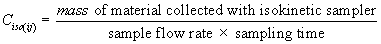where i = replicate number and j = isokinetic sampler number.

(viii) Calculate and record the mean mass concentration as: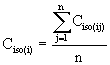where n = total number of isokinetic samplers.

(ix) Calculate and record the coefficient of variation of the mass concentration measurements as: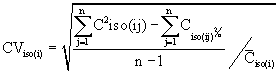If the value of CViso(i) exceeds 0.10, the particle concentration uniformity is unacceptable and steps (vi) through (ix) must be repeated. If adjustment of the vibrating orifice aerosol generator or changes in the particle delivery system are necessary to achieve uniformity, steps (ii) through (ix) must be repeated. Remove the array of isokinetic samplers from the wind tunnel. NOTE: A single isokinetic sampler, operated at the same nominal flow rate as the test sampler, may be used in place of the array of isokinetic samplers for the determination of particle mass concentration used in the calculation of sampling effectiveness of the test sampler in step (xiii). In this case, the array of isokinetic samplers must be used to demonstrate particle concentration uniformity prior to the replicate measurements of sampling effectiveness.

(x) If a single isokinetic sampler is used, install the sampler in the wind tunnel with the sampler nozzle centered in the sampling zone (see § 53.42(d)). Collect particles on an appropriate filter (e.g., glass fiber) for a time period such that the relative error of the measured concentration (as defined in step (vi)) is less than 5 percent. Determine the quantity of material collected with the isokinetic sampler using a calibrated fluorometer. Calculate and record the mass concentration as Ciso(i) as in step vii. Remove the isokinetic sampler from the wind tunnel.

(xi) Install the test sampler (or portion thereof) in the wind tunnel with the sampler inlet opening centered in the sampling zone (see § 53.42(d)). To meet the maximum blockage limit of § 53.42(a) or for convenience, part of the test sampler may be positioned external to the wind tunnel provided that neither the geometry of the sampler nor the length of any connecting tube or pipe is altered. Collect particles on an appropriate filter or filters (e.g., glass fiber) for a time period such that the relative error of the measured concentration (as defined in step (vi)) is less than 5 percent.

(xii) Determine the quantity of material collected with the test sampler using a calibrated fluorometer. Calculate and record the mass concentration as: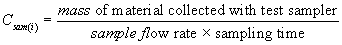where i = replicate number.

(xiii) Calculate and record the sampling effectiveness of the test sampler as: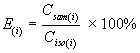where i = replicate number. Note:

If a single isokinetic sampler is used for the determination of particle mass concentration, replace Ciso(i) with Ciso(i).

(xiv) Remove the test sampler from the wind tunnel. Repeat steps (vi) through (xiii), as appropriate, to obtain a minimum of three replicate measurements of sampling effectiveness.

(xv) Calculate and record the average sampling effectiveness of the test sampler as: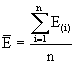where n = number of replicates.

(xvi) Calculate and record the coefficient of variation for the replicate sampling effectiveness measurements of the test sampler as: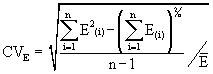If the value of CVE exceeds 0.10, the test run (steps (ii) through (xvi)) must be repeated.

(xvii) Repeat steps i through xvi for each wind speed, particle size, and particle type specified in table D-2.

(xviii) For each of the three wind speeds (nominally 2, 8, and 24 km/hr), correct the liquid particle sampling effectiveness data for the presence of multiplets (doublets and triplets) in the test particle atmospheres.

(xix) For each wind speed, plot the corrected liquid particle sampling effectiveness of the test sampler (Ecorr) as a function of particle size (dp) on semi-logarithmic graph paper where dp is the particle size established by the operating parameters of the vibrating orifice aerosol generator. Construct a smooth curve through the data.

(xx) For each wind speed, calculate the expected mass concentration for the test sampler under the assumed particle size distribution and compare it to the mass concentration predicted for the ideal sampler, as follows:

(A) Extrapolate the upper and lower ends of the corrected liquid particle sampling effectiveness curve to 100 percent and 0 percent, respectively, using smooth curves. Assume that Ecorr = 100 percent at a particle size of 1.0 µm and Ecorr = 0 percent at a particle size of 50 µm.

(B) Determine the value of Ecorr at each of the particle sizes specified in the first column of table D-3. Record each Ecorr value as a decimal between 0 and 1 in the second column of table D-3.

(C) Multiply the values of Ecorr in column 2 by the interval mass concentration values in column 3 and enter the products in column 4 of table D-3.

(D) Sum the values in column 4 and enter the total as the expected mass concentration for the test sampler at the bottom of column 4 of table D-3.

(E) Calculate and record the percent difference in expected mass concentration between the test sampler and the ideal sampler as: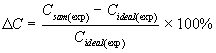where: Csam(exp) = expected mass concentration for the test sampler, µg/m 3 Cideal(exp) = expected mass concentration for the ideal sampler, µg/m 3 (calculated for the ideal sampler and given at the bottom of column 7 of table D-3.)

(F) The candidate method passes the liquid particle sampling effectiveness test if the Δ C value for each wind speed meets the specification in table D-1.

(xxi) For each of the two wind speeds (nominally 8 and 24 km/hr), calculate the difference between the average sampling effectiveness value for the 25 µm solid particles and the average sampling effectiveness value for the 25 µm liquid particles (uncorrected for multiplets).

(xxii) The candidate method passes the solid particle sampling effectiveness test if each such difference meets the specification in table D-1.

Table D-3 - Expected Mass Concentration for PM 10 Samplers

Particle size (um) Test sampler Ideal Sampler
Sampling
effectiveness
Interval mass concentration (µg/m 3) Expected mass concentration (µg/m 3) Sampling
effectiveness
Interval mass concentration (µg/m 3) Expected mass concentration (µg/m 3)
(1) (2) (3) (4) (5) (6) (7)
<1.0 1.000 62.813 62.813 1.000 62.813 62.813
1.5 9.554 0.949 9.554 9.067
02.0 2.164 0.942 2.164 2.038
02.5 1.785 0.933 1.785 1.665
03.0 2.084 0.922 2.084 1.921
03.5 2.618 0.909 2.618 2.380
04.0 3.211 0.893 3.211 2.867
04.5 3.784 0.876 3.784 3.315
05.0 4.300 0.857 4.300 3.685
05.5 4.742 0.835 4.742 3.960
06.0 5.105 0.812 5.105 4.145
06.5 5.389 0.786 5.389 4.236
07.0 5.601 0.759 5.601 4.251
07.5 5.746 0.729 5.746 4.189
08.0 5.834 0.697 5.834 4.066
08.5 5.871 0.664 5.871 3.898
09.0 5.864 0.628 5.864 3.683
09.5 5.822 0.590 5.822 3.435
10.0 5.750 0.551 5.750 3.168
10.5 5.653 0.509 5.653 2.877
11.0 8.257 0.465 8.257 3.840
12.0 10.521 0.371 10.521 3.903
13.0 9.902 0.269 9.902 2.664
14.0 9.250 0.159 9.250 1.471
15.0 8.593 0.041 8.593 0.352
16.0 7.948 0.000 7.948 0.000
17.0 7.329 0.000 7.329 0.000
18.0 9.904 0.000 9.904 0.000
20.0 11.366 0.000 11.366 0.000
22.0 9.540 0.000 9.540 0.000
24.0 7.997 0.000 7.997 0.000
26.0 6.704 0.000 6.704 0.000
28.0 5.627 0.000 5.627 0.000
30.0 7.785 0.000 7.785 0.000
35.0 7.800 0.000 7.800 0.000
40.0 5.192 0.000 5.192 0.000
45.0 4.959 0.000 4.959 0.000
Csam(exp) = D Cideal(exp) = 143.889

(b) 50 Percent cutpoint - (1) Technical definition. The particle size for which the sampling effectiveness of the sampler is 50 percent.

(2) Test procedure. (i) From the corrected liquid particle sampling effectiveness curves for each of the three wind speeds, determine the particle size at which the curve crosses the 50 percent effectiveness line and record as D50 on the corresponding sampling effectiveness plot.

(ii) The candidate method passes the 50 percent cutpoint test if the D50 value at each wind speed meets the specification in table D-1.

(c) Precision - (1) Technical definition. The variation in the measured particle concentration among identical samplers under typical sampling conditions.

(2) Test procedure. (i) Set up three identical test samplers at the test site in strict accordance with the instructions in the manual referred to in § 53.4(b)(3). Locate the test sampler inlet openings at the same height and between 2 and 4 meters apart. The samplers shall be oriented in a manner that will minimize spatial and wind directional effects on sample collection. Perform a flow calibration for each test sampler in accordance with the instructions given in the instruction manual and/or appendix J to part 50 of this chapter. Set the operating flow rate to the value prescribed in the sampler instruction manual.

Note:

For candidate equivalent methods, this test may be used to satisfy part of the requirements of subpart C of this chapter. In that case, three reference method samplers are also used at the test site, measurements with the candidate and reference methods are compared as specified in § 53.34, and the test site must meet the requirements of § 53.30(b).

(ii) Measure the PM 10 concentration of the atmosphere using the three test samplers for 10 periods (test days) of 24 hours each. On each of the 10 test days, measure the initial and final flow rates of each test sampler. On three of the test days, measure the flow rate of each test sampler after 6, 12, and 18 hours of operation. All measurements of flow rate and mass collected must be made in accordance with the procedures prescribed in the sampler instruction manual and/or appendix J to part 50 of this chapter. All measurements of flow rate must be in actual volumetric units. Record the PM 10 concentration for each sampler and each test day as C(i)(j) where i is the sampler number and j is the test day.

(iii) For each test day, calculate and record the average of the three measured PM 10 concentrations as C(j) where j is the test day. If C(j)<30 µg/m 3 for any test day, data from that test day are unacceptable and the tests for that day must be repeated.

(iv) Calculate and record the precision for each of the 10 test days as:(v) The candidate method passes the precision test if all 10 Pj or RPj values meet the specifications in table D-1.

(d) Flow rate stability - (1) Technical definition. Freedom from variation in the operating flow rate of the sampler under typical sampling conditions.

(2) Test procedure. (i) For each of the three test samplers and each of the 10 test days of the precision test, record each measured flow rate as F(i)(j)(t), where i is the sampler number, j is the test day, and t is the time of flow rate measurement (t = 0, 6, 12, 18, or 24 hours).

(ii) For each sampler and for each test day, calculate and record the average flow rate as:where n = number of flow rate measurements during the 24-hour test day.

(iii) For each sampler and for each test day, calculate and record the percent difference between the average flow rate and the initial flow rate as:where F(i)(j)(0) is the initial flow rate (t = 0).

(iv) For each sampler and for each of the 3 test days on which flow measurements were obtained at 6-hour intervals throughout the 24-hour sampling period, calculate and record the percent differences between each measured flow rate and the initial flow rate as:where t = 6, 12, 18, or 24 hours.

(v) The candidate method passes the flow rate stability test if all of the Δ F(i)(j) and Δ F(i)(j)(t) values meet the specifications in table D-1.